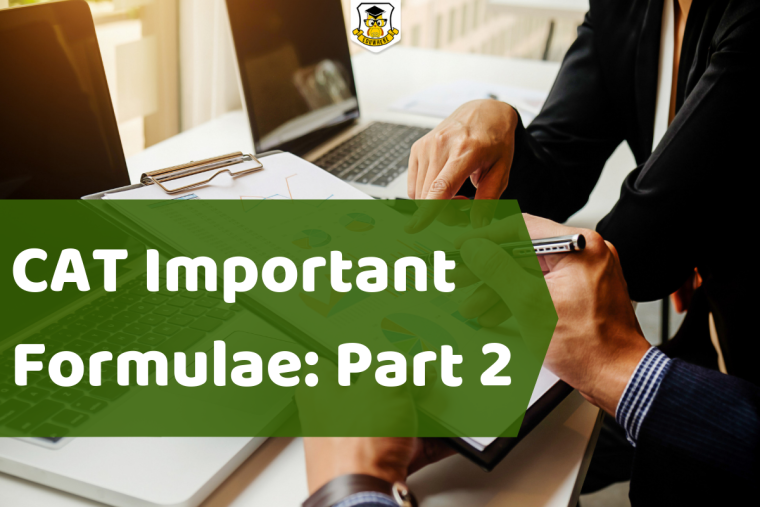## CAT Important Formulae: Part 2Progressions (AP, HP, GP)

When a set of numbers arranged in such a way that it forms a series and the next number can be predicted from the series, it is called as Progression.

Progression related problems are asked in the CAT examination in the Quantitative Aptitude section.

If the difference between 2 consecutive terms is always the same, it is called Arithmetic Progression. Hence, the common difference between the numbers is considered for an Arithmetic Progression.

For Example 3, 6,  9, 12, 15 is an AP.

If ‘a’ is the first term and 'd’ is a common difference, nth term of an AP = a + (n-1) d

Arithmetic Mean = Sum of all terms in the AP / Number of terms in the AP

Sum of ‘n’ terms of an AP = 0.5 n (first term + last term) = 0.5 n [ 2a + (n-1) d ]

Geometric Progression (GP)

In a case where the ratio of any two consecutive terms is always the same, it is called Geometric Progression. In this series, the next number is calculated by multiplying a common number to the previous number.

For Example 4, 8, 16, 32

If ‘a’ is the first term and ‘r’ is the common ratio, nth term of a GP = a rn-1

Geometric Mean = nth root of the product of n terms in the GP

Sum of ‘n’ terms of a GP (r < 1) = [a (1 – rn)] / [1 – r]

Sum of ‘n’ terms of a GP (r > 1) = [a (rn – 1)] / [r – 1]

Sum of infinite terms of a GP (r < 1) = (a) / (1 – r)

Harmonic Progression (HP)

When the reciprocal of the terms are in AP, it is called a Harmonic Progression.

Let’s consider 2 terms ‘a’ and ‘b’.

Harmonic Mean for the same will be (2 a b) / (a + b).

Let’s consider there are two numbers. For them, if A, G, and H are respectively the arithmetic, geometric and harmonic means, then A ≥ G ≥ H

A H = G2, i.e., A, G, H are in GP

1

2235